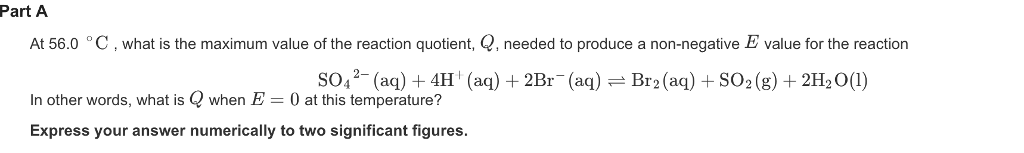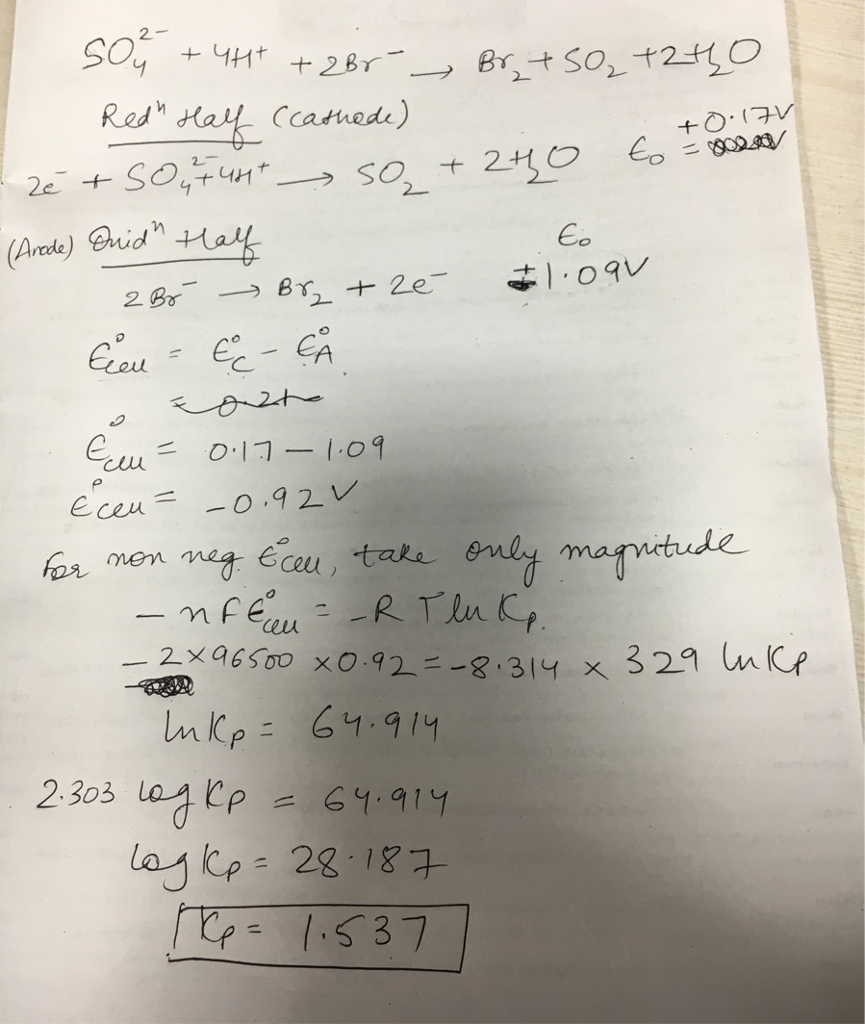# Question & Answer: At 56.0 degree C, what is the maximum value of the reaction quotient, Q, needed to produce a…..At 56.0 degree C, what is the maximum value of the reaction quotient, Q, needed to produce a non-negative E value for the reaction SO^2-_4(aq) + 4H^+(aq) + 2Br^-(aq) doubleheadarrow Br_2(aq) + SO_2(g) + 2H_2O(l) In other words, what is Q when E = 0 at this temperature? Express your answer numerically to two significant figures.